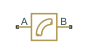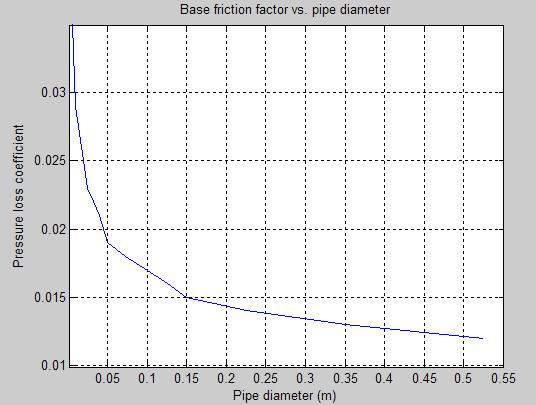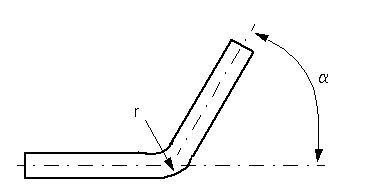# Pipe Bend

(To be removed) Hydraulic resistance in pipe bend

The Hydraulics (Isothermal) library will be removed in a future release. Use the Isothermal Liquid library instead. (since R2020a)

## Library

Local Hydraulic Resistances

•## Description

The Pipe Bend block represents a pipe bend as a local hydraulic resistance. The pressure loss in the bend is assumed to consist of

• Loss in the straight pipe

• Loss due to curvature

The loss in a straight pipe is simulated with the Hydraulic Resistive Tube block. The loss due to curvature is simulated with the Local Resistance block, and the pressure loss coefficient is determined in accordance with the Crane Co. recommendations (see , p. A-29). The flow regime is checked in the underlying Local Resistance block by comparing the Reynolds number to the specified critical Reynolds number value.

The pressure loss due to curvature for turbulent flow regime is determined according to the following formula:

`$p=K\frac{\rho }{2{A}^{2}}q|q|$`

where

 `q` Flow rate `p` Pressure loss `K` Pressure loss coefficient `A` Bend cross-sectional area ρ Fluid density

For laminar flow regime, the formula for pressure loss computation is modified, as described in the reference documentation for the Local Resistance block.

The pressure loss coefficient is determined according to recommendation provided in :

`$K={K}_{d}·{K}_{r}·{K}_{\alpha }$`

where

 `Kd` Base friction factor coefficient `Kr` Correction coefficient accounting for the bend curvature `K`α Correction coefficient accounting for the bend angle

The base friction factor coefficient is determined according to the following table.Note

Friction factors for pipes with diameters greater than 525 mm are determined by extrapolation.

The correction coefficient accounting for the bend curvature is determined according to the next table.The bend curvature relative radius is calculated as

`r` = `bend radius` / `pipe diameter`

Note

For pipes with the bend curvature relative radius value outside the range of 1 > `r` > 24, correction coefficients are determined by extrapolation.

Correction for non-90o bends is performed with the empirical formula (see , Fig. 4.6):

`${K}_{\alpha }=\alpha \left(0.0142-3.703·{10}^{-5}\alpha \right)$`

where

 α Bend angle in degrees (0 ≤ α ≤ 180)Connections A and B are conserving hydraulic ports associated with the block inlet and outlet, respectively.

The block positive direction is from port A to port B. This means that the flow rate is positive if fluid flows from A to B, and the pressure differential is determined as $p={p}_{A}-{p}_{B}$.

Warning

The formulas used in the Pipe Bend block are very approximate, especially in the laminar and transient flow regions. For more accurate results, use a combination of the Local Resistance block with a table-specified `K=f(Re)` relationship and the Hydraulic Resistive Tube block.

## Basic Assumptions and Limitations

• Fluid inertia and wall compliance are not taken into account.

• The bend is assumed to be made of a clean commercial steel pipe.

## Parameters

Pipe diameter

The internal diameter of the pipe. The default value is `0.01` m.

The radius of the bend. The default value is `0.04` m.

Bend angle

The angle of the bend. The value must be in the range between 0 and 180 degrees. The default value is `90` deg.

Internal surface roughness height

Roughness height on the pipe internal surface. The parameter is typically provided in data sheets or manufacturer's catalogs. The default value is `1.5e-5` m, which corresponds to drawn tubing.

Critical Reynolds number

The maximum Reynolds number for laminar flow. The value of the parameter depends on the orifice geometrical profile. You can find recommendations on the parameter value in hydraulics textbooks. The default value is `350`.

Fluid compressibility

Dynamic compressibility setting. Select `On` to make the fluid density dependent on pressure and temperature. Select `Off` to treat the fluid density as a constant. Dynamic compressibility impacts the transient response of the fluid at small time scales and can slow down simulation.

Initial liquid pressure (gauge)

Gauge pressure in the pipe bend at time zero. The default value is `0` Pa.

## Global Parameters

Parameters determined by the type of working fluid:

• Fluid density

• Fluid kinematic viscosity

Use the Hydraulic Fluid block or the Custom Hydraulic Fluid block to specify the fluid properties.

## Ports

The block has the following ports:

`A`

Hydraulic conserving port associated with the bend inlet.

`B`

Hydraulic conserving port associated with the bend outlet.

## References

 Flow of Fluids Through Valves, Fittings, and Pipe, Crane Valves North America, Technical Paper No. 410M

 George R. Keller, Hydraulic System Analysis, Published by the Editors of Hydraulics & Pneumatics Magazine, 1970

## Version History

Introduced in R2006b

expand all